##### Pre-Calculus For Dummies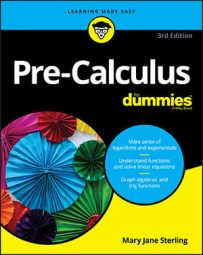Polar coordinates are an extremely useful addition to your mathematics toolkit because they allow you to solve problems that would be extremely ugly if you were to rely on standard x- and y-coordinates. In order to fully grasp how to plot polar coordinates, you need to see what a polar coordinate plane looks like.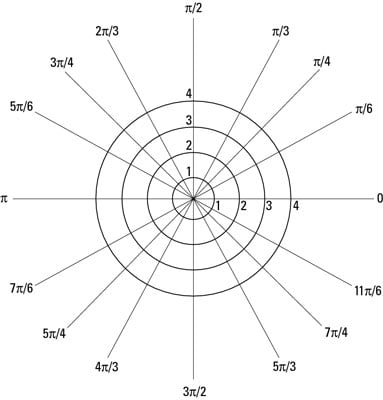A blank polar coordinate plane (not a dartboard)
In the figure, you can see that the plane is no longer a grid of rectangular coordinates; instead, it's a series of concentric circles around a central point, called the pole. The plane appears this way because the polar coordinates are a given radius and a given angle in standard position from the pole. Each circle represents one radius unit, and each line represents the special angles from the unit circle.

Because you write all points on the polar plane as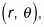to graph a point on the polar plane, you should find theta first and then locate r on that line. This approach allows you to narrow the location of a point to somewhere on one of the lines representing the angle. From there, you can simply count out from the pole the radial distance. If you go the other way and start with r, you may find yourself in a pickle when the problems get more complicated.

## Plotting polar coordinates example

For example, to plot point E at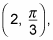which has a positive value for both the radius and the angle — you simply move from the pole counterclockwise until you reach the appropriate angle (theta). You start there in the following list:

1. Locate the angle on the polar coordinate plane.

Refer to the figure to find the angle: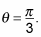2. Determine where the radius intersects the angle.

Because the radius is 2 (r = 2), you start at the pole and move out 2 spots in the direction of the angle.

3. Plot the given point.

At the intersection of the radius and the angle on the polar coordinate plane, plot a dot and call it a day! This figure shows point E on the plane.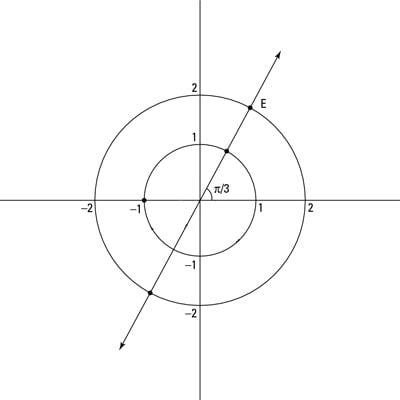Visualizing simple and complex polar coordinates
Polar coordinate pairs can have positive angles or negative angles for values of theta. In addition, they can have positive and negative radii. This concept might be new; in past classes, you might've always heard that a radius must be positive. When graphing polar coordinates, though, the radius can be negative, which means that you move in the opposite direction of the angle from the pole.

Because polar coordinates are based on angles, unlike Cartesian coordinates, polar coordinates have many different ordered pairs. Because infinitely many values of theta have the same angle in standard position, an infinite number of coordinate pairs describe the same point. Also, a positive and a negative co-terminal angle can describe the same point for the same radius, and because the radius can be either positive or negative, you can express the point with polar coordinates in many ways.